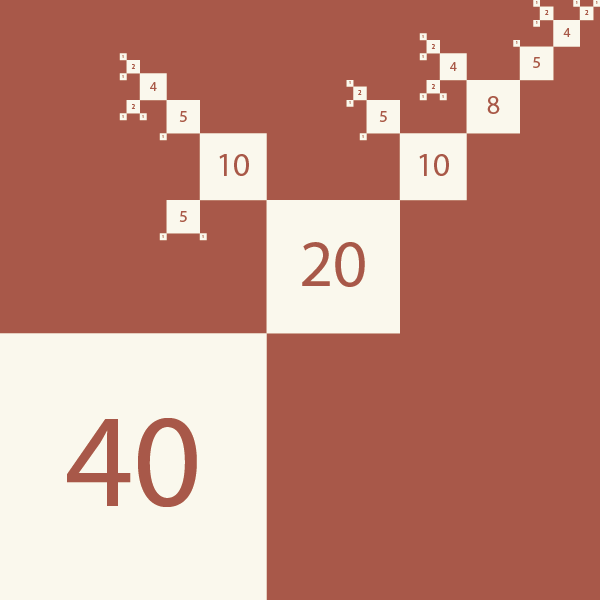# Ample andpristine numbers

Number theory

Parallels between the perfect and abundant numbers and their recursive analogs point to deeper structure in the recursive divisor function.

## Recursively abundant and recursively perfect numbers

Arxiv (2020)

T. Fink

The divisor function $\sigma(n)$ sums the divisors of $n$. We call $n$ abundant when $\sigma(n) - n > n$ and perfect when $\sigma(n) - n = n$. I recently introduced the recursive divisor function $a(n)$, the recursive analog of the divisor function. It measures the extent to which a number is highly divisible into parts, such that the parts are highly divisible into subparts, so on. Just as the divisor function motivates the abundant and perfect numbers, the recursive divisor function motivates their recursive analogs, which I introduce here. A number is recursively abundant if $a(n) > n$ and recursively perfect if $a(n) = n$. There are striking parallels between abundant and perfect numbers and their recursive counterparts. The product of two recursively abundant numbers is recursively abundant, and recursively abundant numbers are either abundant or odd perfect numbers. Odd recursively abundant numbers exist but are rare, and I conjecture that there are such numbers not divisible by the first $k$ primes—which is known to be true for the abundant numbers. There are infinitely many recursively perfect numbers, but that they cannot be odd, apart from 1. Recursively perfect numbers are the product of a power of two and odd prime solutions to certain Diophantine equations, reminiscent of how perfect numbers are the product of a power of two and a Mersenne prime.

Arxiv (2020)

T. Fink